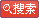# GMATЪ§бЇ--ЫуЪѕИХЪіЃЈЖўЃЉ

ЗЂВМЪБМфЃК2007-9-3ЁЁЮФзжДѓаЁЃКДѓ жа аЁЁЁДђгЁЃКДђгЁДЫЮФ

Жў.ЗжЪ§ЃКfractions

ЗжзгЃКnumerator

ЗжФИЃКdenominator

ЗжЪ§ЕФМгМѕГЫГ§ЃКadditionЃЌsubtractionЃЌmultiplication and division of fractions

ЗБЗжЪ§КЭМйЗжЪ§ЃКmixed number and improper fraction

ЗБЗжЪ§ЪЧжИвЛИіЪ§гЩвЛИіећЪ§КЭвЛИіЗжЪ§ЙЙГЩЁЃ

МйЗжЪ§ЪЧжИЗжзгДѓгкЗжФИЕФЗжЪ§ЁЃР§ШчЃК7/3

Ш§.аЁЪ§ЃКdecimals

ПЦбЇМЦЪ§ЗЈЃКscientific notation

Sometimes decimals are expressed as the product of a number with only one digit to the left of the decimal point and a power of 10. This is called scientific notation. For example, 231 can be written as 2.31ЁС102 and 0.0231 can be written as 2.31ЁС10-2. When a number is expressed in scientific notation, the exponent of the

10 indicates the number of places that the decimal point is to be moved in the number that is to be multiplied by a power of 10 in order to obtain the product. The decimal point is moved to the right if the exponent is positive and to the left if the exponent is negative. For example, 20.13ЁС103 is equal to 20,130 and 1.91ЁС10-4 is equal to 0.000191.

ЫФЩсЮхШыЃКto the nearest

аЁЪ§ЕуЃКdecimal pointЃЌperiod

ЫФ.ЪЕЪ§ЃКreal numbers

е§Ъ§КЭИКЪ§ЃКpositive and negative numbers

ОјЖджЕЃКabsolute value

Юх.БШТЪгыБШР§ЃКratio and proportion

вЛИіБШТЪratioПЩвдБэЪОГЩаэЖрЗНЪНЃЌР§ШчЃКthe ratio of 2 to 3ПЩвдБЛБэДяЮЊ2 to 3ЃЌ2ЃК3ЃЌЛђеп2/3ЁЃзЂвтБШТЪжаЕФжаЯюЕФЫГађЪЧживЊЕФЃЌМД2 to 3КЭ3 to 2ВЛЭЌЁЃA proportion isa

statement that two ratios are equalЁЃР§ШчЃК2/3=8/12ЪЧвЛИіproportionЁЃ

Сљ.АйЗжБШЃКpercent

Percent means per hundred or number out of 100ЁЃ

дкПМЬтжаО­ГЃЛсЮЪЕНДгФГвЛЪ§СПЕНСэвЛЪ§СПАйЗжБШЕФдіМгЛђМѕЩйЁЃЪзЯШЫуГідіМгЛђМѕЩйЕФСПЃЌШЛКѓГ§вдд­РДЕФФЧИіСПЃЌМДЁАfromЁБЛђЁАthanЁБКѓУцЕФСПЁЃ

Цп.Ъ§ЕФУнКЭИљЃКpowers and roots of numbers

x nвтЮЖзХthe nth power of xЁЃР§ШчЃК64 is the 6th power of 2ЁЃ2 is a 6th root of 64ЁЃ

СЂЗНИљЪЧжИcube rootЁЃ

ЮФеТЃКЁАGMATЪ§бЇ--ЫуЪѕИХЪіЃЈЖўЃЉЁБе§ЮФЭъ
ШШЕуЮФеТ
дкЯпзЩбЏ
ЁЁ